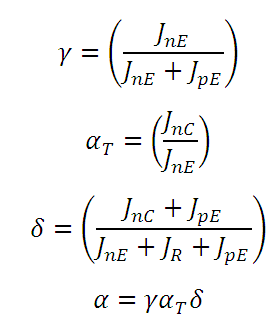# Emitter injection efficiency factor, Base Transport Factor, Recombination factor of a BJT

This CalcTown calculator calculates the emitter injection efficiency factor, base Transport Factor and recombination factor of a BJT.

cm-3
cm-3
cm-3
cm-3

#### Resultwhere

αT=Base transport Factor

δ=Recombination factor

λ=Emitter injection Efficiency Factor

α=Common Base Current Gain